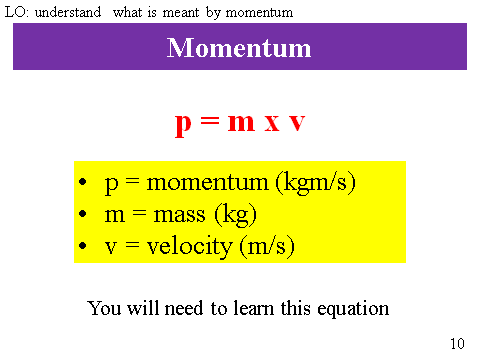# Momentum

## Momentum

Momentum is a measure of the mass and velocity of an object. A massive object and a tiny object can both have the same momentum, but the larger object must be travelling very slowly and the smaller object travelling really quickly.

## Momentum Equation

You need to recall and apply this equation. Example:Calculate the momentum of an athlete of mass 60 kg running at a velocity of 10 m/s?

p = m x v

p = 60 x 10 = 600kgm/s

## 5Mark Questions

Momentum is an easy topic to create five mark questions.

This is because we already know a number of equations that include mass and velocity!

Task: Write down all the the equations in this topic that include mass and velocity so you can see how it fits into momentum.

Calculate the momentum of a mouse of mass 500g scuttling through the grass at 3m/s